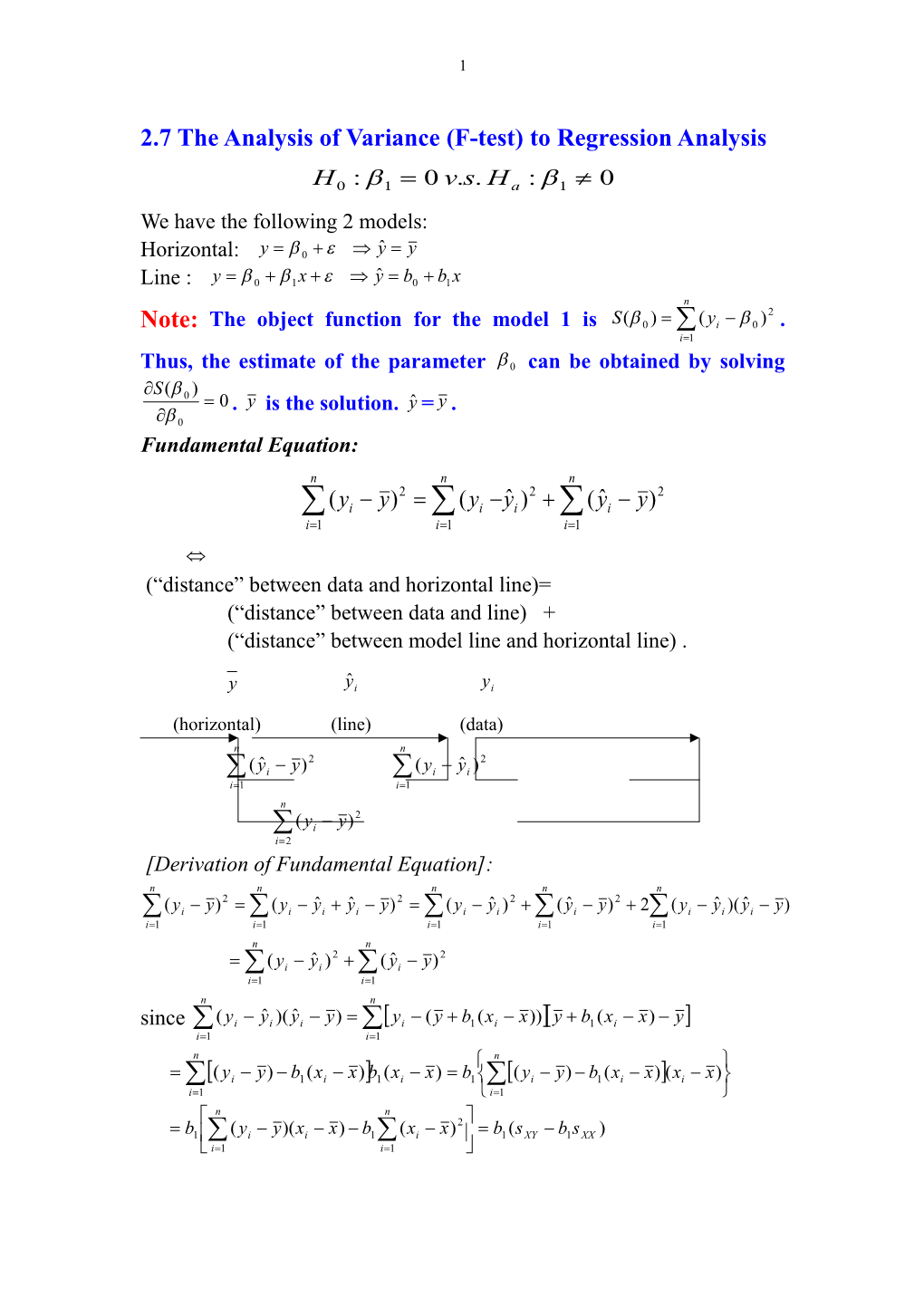# 2.7 the Analysis of Variance (F-Test) to Regression Analysis1

2.7 The Analysis of Variance (F-test) to Regression Analysis

We have the following 2 models:

Horizontal:

Line :

Note:The object function for the model 1 is . Thus, the estimate of the parameter can be obtained by solving . is the solution. =.

Fundamental Equation:

(“distance”between data and horizontal line)=

(“distance” between data and line) +

(“distance” between model line and horizontal line) .

(horizontal) (line) (data)

[Derivation of Fundamental Equation]:

since

The ANOVA (Analysis of Variance) table corresponding to the fundamental equation:

Source / df / SS / MS
Due to regression
/ 1 / /
Residual (Error) / n-2 / /
Total (corrected) / n-1 /

Let

,

the ratio of the mean sum of squares due to the regression and mean residual sum of squares. Intuitively, large F value might imply the difference between the line and the horizontal line is relatively large to the random variation reflected by the mean residual sum of squares. That is, is so significant such that the difference between the line and the horizontal line are apparent. Therefore, the F value can provide important information about if .

Next question to ask: how large value of F can be considered to be large? To test ,

Note:The sum ofsquares due to the regression and the mean sum of squares due to regression are .

The total sum of squares is

Thus, the f statistic is .

Note:For ease of computation, the following equations can be used:

.

Note:.

Note:Let t be the statistic for testing . Then, .

Motivating Example (continue):

Assume . To test , we have the following:

Thus, we have the following ANOVA table

Source / df / SS / MS / f
Regression / 1 / SSR=14200 / /
Residual (Error) / n-2=8 / SSE=1530 /
Total (corrected) / 9 / 15730

Since

,

we reject . Note that

.

Example 2 (continue):

Suppose the model is

,

and

(a)Provide an ANOVA table.

(b)Find the 95% confidence interval for .and use the confidence interval to test .

[solution:]

(a)

Since

The ANOVA table is

Source / df / SS / MS
Residual (Error) / n-2=18 / SSE=3.848 /
Regression / 1 / SSR=49.220 /
Total (corrected) / 19 / 53.068

(b) The 95% confidence interval for is

.

Since , we reject .

Example 3:

Given are 5 observations for two variables x and y.

/ 2 / 3 / 5 / 1 / 8
/ 25 / 25 / 20 / 30 / 16

Suppose the model is

,

(a)Find the least square estimate and the fitted regression equation

(b)Provide an ANOVA table and use F statistic to test at .

(c) Use t statistic to test at .

(d)Find the 95% confidence interval for .and use the confidence interval to test .

[solutions:]

(a)Since

thus,

Then, the least square estimate is

The fitted regression equation is

.

(b)

Since

The ANOVA table is

Source / df / SS / MS / F
Regression / 1 / SSR=108.467 / /
Residual (Error) / n-2=3 / SSE=6.333 /
Total (corrected) / n-1=4 / SST=114.8

Since , we reject .

(c)

.

Since

,

we do not reject .

(d)

The 95% confidence interval for is

.

Since , we do not reject .